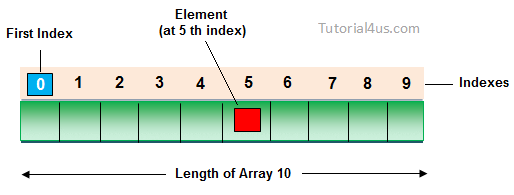# Array in C++

An array is a collection of similar data type value in a single variable. It is a derived data type in C++, which is constructed from fundamental data type of C++ language.• Code Optimization: Less code is required, one variable can store numbers of value.
• Easy to traverse data: By using array easily retrieve the data of array.
• Easy to sort data: Easily short the data using swapping technique
• Random Access: With the help of array index you can randomly access any elements from array.

Fixed Size: Whatever size, we define at the time of declaration of array, we can not change their size, if you need more memory in that time you can not increase memory size, and if you need less memory in that case also wastage of memory.

### Declaring Array

To declare an array in C++ required type of array elements and size of array.

## Syntax

`Datatype arrayName[SIZE];`

## Initializing Array

Initializing is a process to initialize the value in array variable. This is happen in two ways, initialize array one by one or all elements are initializing once.

## Initialization of array one by one

```int arr;
arr=10;
arr=20;
arr=30;
arr=40;
arr=50;```

## Initialization of array at once

`int arr[]={10,20,30,40,50};`

### Accessing Array Elements in C++

We can access array elements with the help of index value of element.

## Example of Array in C++

```#include<iostream.h>
#include<conio.h>

void main()
{
int i, marks[]={80, 62, 70, 90, 98}; //declaration and initialization of array
clrscr();

//traversal of array
for(i=0;i<5;i++)
{
cout<<endl<<marks[i];
}
getch();
}```

```
80
62
70
90
98
```

## 2-dimentional array

• In 2-dimentional elements are arranged in row and column format.
• When we are working with 2-dimentional array we require to refer 2-subscript operator which indicates row and column sizes.
• The main memory of 2-dimentional array is rows and sub-memory is columns.
• On 2-dimentional array when we are referring one-subscript operator then if gives row address, 2-subscript operator will gives element.
• On 2-dimentional array arrayName always gives main memory that is 1st row base address, arrayName will gives next row base address.

## Syntax

`Datatype  ArrayName[SIZE][SIZE];`

### Important points related to array

Always size of the array must be an unsigned integer value which is greater than ‘0’ only. In declaration of the array size must be required to mention, if size is not mention then compiler will give an error.

## Example

`int arr[];	//error`

In declaration of the array size must be assigned type which value is greater than 0. In initialization of the array if specific number of values are not initialized it then rest of all elements will be initialized with it ‘0’.

## Example

```int arr={10,20};	// yes valid
arr=10;
arr=20;
arr, arr, arr;	// initialized with zero```

In initialization of the array mentioning the size is optional, in this case how many elements are initialize it that many variable are created.

## Example of Array in C++

```int arr[]={10,20,30,40,50};	// valid
Size=5;
Sizeof(arr)=10 byte```

## Important points for Array

• In implementation when we required ‘n’ number of variables of same data type then go for an array.
• When we are working with arrays always memory will created in continues memory location, so randomly we can access the data.
• In arrays all elements will share same name with unique identification value called index.
• Always array index will start with ‘0’ and end with ‘size-1’.
• When we are working with array compile time memory management will occur that is static memory allocation.